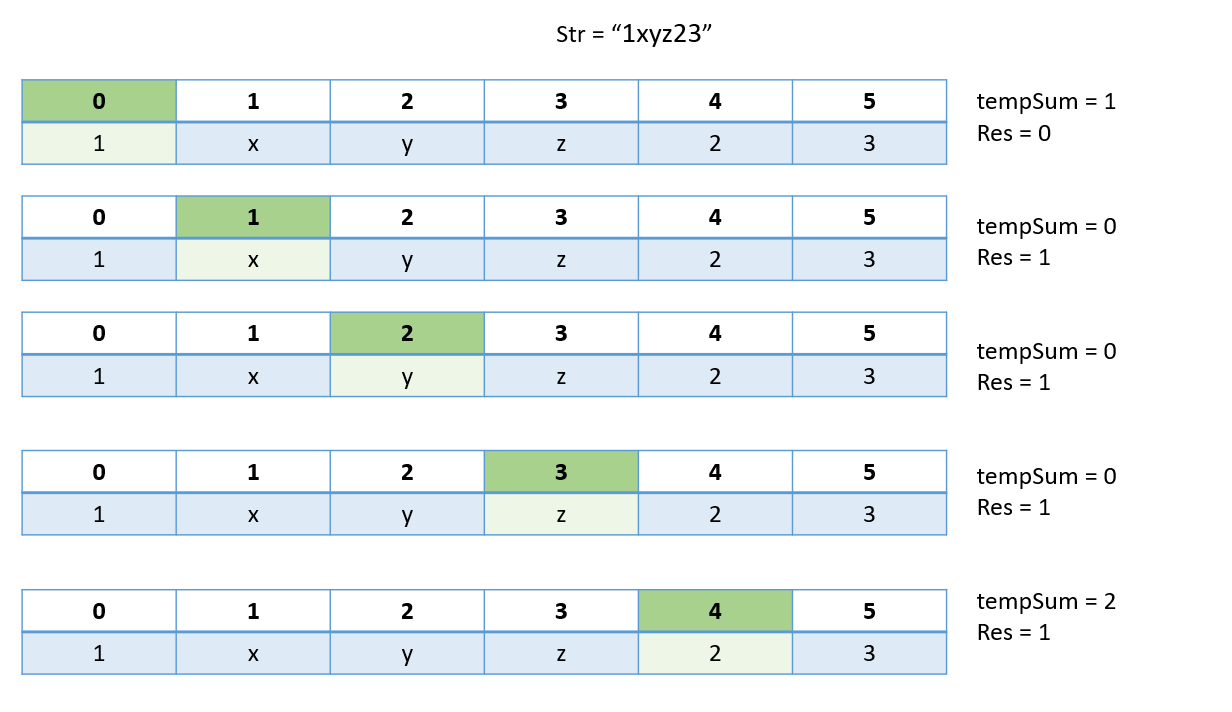# Sum of the Numbers in a String

Problem: Given a string, calculate the sum of numbers in a string (multiple consecutive digits are considered one number)

Examples:

```Example 1:
Input: string = “123xyz”
Output: 123

Example 2:
Input: string = “1xyz23”
Output: 24
```

### Solution

Disclaimer: Don’t jump directly to the solution, try it out yourself first.

Intuition :

• We will iterate in the whole string, if we encounter consecutive numbers we will store them in a temporary string.
• After the end of consecutive numbers, we will add them to our final result. This process goes on till we reach the end of the string.
• At the end, we will print the sum.

Approach:

• We will use a tempSum string to store the number
• Using a for loop we will iterate through the string and if we encounter a number we will add this number to our tempSum.
• If we encounter an alphabet , we will add the value of tempSum to the final result and empty the tempSum to get a new number in the string.
• Finally we print the result.Code:

## C++ Code

``````#include <bits/stdc++.h>
using namespace std ;

class Solution {
public:

int sumOfIntegers(string &st, int l) {

string tempSum = "" ;
int sum = 0  ;
for (int i = 0; i < l; i++) {

if (st[i] >= '0' && st[i] <= '9') {
tempSum += st[i]  ;
}
else {
sum += atoi(tempSum.c_str()) ;
tempSum = ""  ;
}
}
return sum + atoi(tempSum.c_str()) ;
}
} ;
int main() {

string st = "1a30z67"  ;

int l = st.length()  ;

Solution obj ;
cout << "Sum: "  ;
cout << obj.sumOfIntegers(st, l)  ;

return 0  ;
}
``````

Output: Sum: 98

Time Complexity: O(n)

Reason: We make a single iteration to get the result array

Space Complexity: O(n)

Reason: We are storing a new string of length n in the iteration.

## Java Code

``````import java.util.*;

public class Solution {

static int findSum(String str) {
String tempSum = "0";
int sum = 0;
for (int i = 0; i < str.length(); i++) {
char s = str.charAt(i);

if (Character.isDigit(s))
tempSum += s;

else {
sum += Integer.parseInt(tempSum);
tempSum = "0";
}
}

return sum + Integer.parseInt(tempSum);
}

public static void main(String[] args) {

String str = "1bc268";
System.out.print("Sum: ");
System.out.println(findSum(str));
}
}

``````

Output: Sum: 269

Time Complexity: O(n)

Reason: We make a single iteration to get the result array

Space Complexity: O(n)

Reason: We are storing a new string of length n in the iteration.

Special thanks to Shreyas Vishwakarma for contributing to this article on takeUforward. If you also wish to share your knowledge with the takeUforward fam, please check out this article